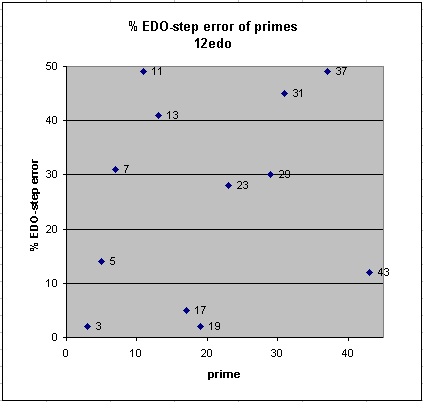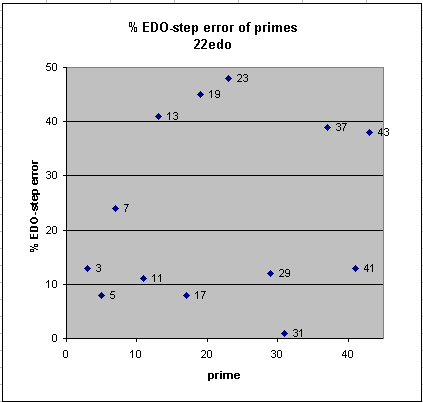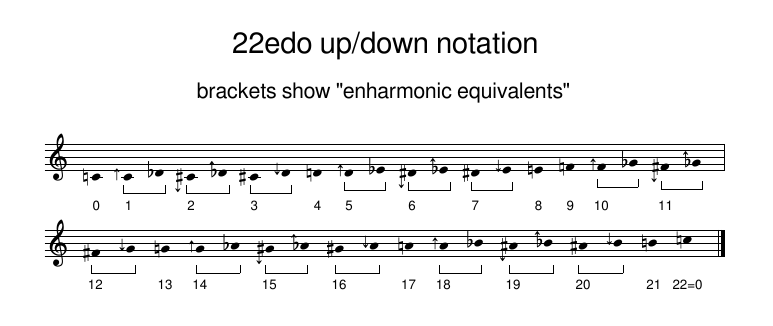# 22-edo

[Joe Monzo]

22-edo (also called 22-ET or 22-tone equal-temperament, when used as a temperament of JI), divides the octave into 22 equal degrees, each having a logarithmic size of exactly 54 + 6/11 (= ~54.54_) cents.

Used as a temperament, 22-edo is a fairly small cardinality which gives a fairly good approximation to 11-limit JI:

• Its best mapping of prime-factor 3 is to 35 degrees of 22-edo (35\22); narrowing that by one octave (i.e., subtracting 22 degrees: 35 - 22 = 13), its mapping of the perfect-5th of 3:2 ratio is to 13 degrees of 22-edo (13\22), which equals exactly 709 + 1/11 (= ~709.09_) cents, with an absolute prime-error of ~+7.1 cents and a relative prime-error of +13% of a 22-edo degree.
• Its best mapping of prime-factor 5 is to 51\22; narrowing that by 2 octaves (i.e., subtracting 2 * 22 = 44 degrees: 51 - 44 = 7), its mapping of the just major-3rd of 5:4 ratio is to 7\22, which equals exactly 381 + 9/11 (= ~381.81_) cents, with an absolute prime-error of ~-4.5 cents and a relative prime-error of ~-8% of a 22-edo degree.
• Its best mapping of prime-factor 7 is to 62\22; narrowing that by 2 octaves (i.e., subtracting 2 * 22 = 44 degrees: 62 - 44 = 18), its mapping of the harmonic-7th of 7:4 ratio is to 18\22, which equals exactly 981 + 9/11 (= ~981.81_) cents, with an absolute prime-error of ~13 cents and a relative prime-error of ~24% of a 22-edo degree.
• Its best mapping of prime-factor 11 is to 76\22; narrowing that by 3 octaves (i.e., subtracting 3 * 22 = 66 degrees: 76 - 66 = 10), its mapping of the _ of 11:8 ratio is to 10\22, which equals exactly 545 + 5/11 (= ~545.45_) cents, with an absolute prime-error of ~-5.9 cents and a relative prime-error of -11% of a 22-edo degree.

Note that 22edo's mappings of prime-factors 3 and 7 are larger (wider, positive), while its mappings of 5 and 11 are smaller (narrow, negative) and both of the narrower mappings have less error than the wider mappings. Below is a graphic comparing the absolute prime-error (i.e., not showing whether it is positive or negative) of the familiar 12edo with that of 22edo:One popular method of notating 22-edo on a standard 5-line musical staff is called "up/down notation", using the standard A B C D E F G nominals in such a way that what appears to be a whole-tone as A:B, C:D, D:E, F:G, G:A spans 4 degrees of 22-edo (4\22), and what appears to be a diatonic-semitone as B:C, E:F, or any nominal paired with either a sharp on the note below or a flat on the note above, spans 1 degree of 22-edo (1\22), and employing as additional accidentals up or down arrows, either alone or attached to sharps or flats as necessary. There is an abundance of enharmonic-equivalents in this notation, only the most basic of which are shown in the graphic below:

#### 22edo up/down notation, on standard 5-line musical staffAnother notation system available for 22-edo is sagittal, which operates exactly the same as up/down but employs different accidentals.

Below is a table showing precise values for the mappings of 22-edo in 41-limit JI, with several example ratios and commas:

```=======================================
edomap.py (c)2008-12-23 by Joseph Monzo
=======================================

Finds the EDO mappings for a set of ratios

22 -edo floating-point mappings:

prime  edo-steps   step-error  edo-map

2  =  22.000000    +0.00 -->     22
3  =  34.869175    +0.13 -->     35
5  =  51.082418    -0.08 -->     51
7  =  61.761808    +0.24 -->     62
11  =  76.107496    -0.11 -->     76
13  =  81.409674    -0.41 -->     81
17  =  89.924183    +0.08 -->     90
19  =  93.454405    -0.45 -->     93
23  =  99.518363    +0.48 -->    100
29  = 106.875582    +0.12 -->    107
31  = 108.992319    +0.01 -->    109
37  = 114.607974    +0.39 -->    115
41  = 117.866144    +0.13 -->    118

integer (i.e., true) mappings, compared with cents-value of actual prime

map  2 -->     22 = 1200.000000 cents <-- 1200.000000  + 0.0 cents
map  3 -->     35 = 1909.090909 cents <-- 1901.955001  + 7.1 cents
map  5 -->     51 = 2781.818182 cents <-- 2786.313714  - 4.5 cents
map  7 -->     62 = 3381.818182 cents <-- 3368.825906  +13.0 cents
map 11 -->     76 = 4145.454545 cents <-- 4151.317942  - 5.9 cents
map 13 -->     81 = 4418.181818 cents <-- 4440.527662  -22.3 cents
map 17 -->     90 = 4909.090909 cents <-- 4904.955410  + 4.1 cents
map 19 -->     93 = 5072.727273 cents <-- 5097.513016  -24.8 cents
map 23 -->    100 = 5454.545455 cents <-- 5428.274347  +26.3 cents
map 29 -->    107 = 5836.363636 cents <-- 5829.577194  + 6.8 cents
map 31 -->    109 = 5945.454545 cents <-- 5945.035572  + 0.4 cents
map 37 -->    115 = 6272.727273 cents <-- 6251.344039  +21.4 cents
map 41 -->    118 = 6436.363636 cents <-- 6429.062406  + 7.3 cents

--------------

examples:

ratio         -->      22 -edo mapping:

ratio      cents   error      edo    cents    name

2:1     = 1200.0  + 0.0 -->  22/22 = 1200.0  (octave)

31:16    = 1145.0  + 0.4 -->  21/22 = 1145.5  (31st harmonic)
243:128   = 1109.8  +35.7 -->  21/22 = 1145.5  (pythagorean major-7th)

15:8     = 1088.3  + 2.6 -->  20/22 = 1090.9  (just major-7th, 5*3)

4096:2187  = 1086.3  -50.0 -->  19/22 = 1036.4  (pythagorean diminished-8ve)
13:7     = 1071.7  -35.3 -->  19/22 = 1036.4  (tridecimal superminor-7th)
11:6     = 1049.4  -13.0 -->  19/22 = 1036.4  (undecimal submajor-7th)
20:11    = 1035.0  + 1.4 -->  19/22 = 1036.4  (undecimal superminor-7th)
29:16    = 1029.6  + 6.8 -->  19/22 = 1036.4  (29th harmonic)
9:5     = 1017.6  +18.8 -->  19/22 = 1036.4  (just minor-7th)

16:9     =  996.1  -14.3 -->  18/22 =  981.8  (pythagorean minor-7th)
7:4     =  968.8  +13.0 -->  18/22 =  981.8  (7th harmonic)

12:7     =  933.1  - 5.9 -->  17/22 =  927.3  (septimal supermajor-6th)
27:16    =  905.9  +21.4 -->  17/22 =  927.3  (pythagorean major-6th)

5:3     =  884.4  -11.6 -->  16/22 =  872.7  (just major-6th)
18:11    =  852.6  +20.1 -->  16/22 =  872.7  (undecimal neutral-6th)
6561:4096  =  815.6  +57.1 -->  16/22 =  872.7  (pythagorean augmented-5th)

13:8     =  840.5  -22.3 -->  15/22 =  818.2  (13th harmonic)
8:5     =  813.7  + 4.5 -->  15/22 =  818.2  (just minor-6th)

128:81    =  792.2  -28.5 -->  14/22 =  763.6  (pythagorean minor-6th)
11:7     =  782.5  -18.9 -->  14/22 =  763.6  (undecimal augmented-5th)
25:16    =  772.6  - 9.0 -->  14/22 =  763.6  (just augmented-5th)
14:9     =  764.9  - 1.3 -->  14/22 =  763.6  (septimal subminor-9th)

3:2     =  702.0  + 7.1 -->  13/22 =  709.1  (perfect-5th)

16:11    =  648.7  + 5.9 -->  12/22 =  654.5  (11th subharmonic)
23:16    =  628.3  +26.3 -->  12/22 =  654.5  (23rd harmonic)
729:512   =  611.7  +42.8 -->  12/22 =  654.5  (pythagorean augmented-4th)

10:7     =  617.5  -17.5 -->  11/22 =  600.0  (septimal large-tritone)
7:5     =  582.5  +17.5 -->  11/22 =  600.0  (septimal small-tritone)

1024:729   =  588.3  -42.8 -->  10/22 =  545.5  (pythagorean diminished-5th)
11:8     =  551.3  - 5.9 -->  10/22 =  545.5  (11th harmonic)

4:3     =  498.0  - 7.1 -->   9/22 =  490.9  (perfect-4th)
21:16    =  470.8  +20.1 -->   9/22 =  490.9  (septimal-4th, 7*3)

9:7     =  435.1  + 1.3 -->   8/22 =  436.4  (septimal supermajor-3rd)
41:32    =  429.1  + 7.3 -->   8/22 =  436.4  (41st harmonic)
14:11    =  417.5  +18.9 -->   8/22 =  436.4  (undecimal diminished-4th)
81:64    =  407.8  +28.5 -->   8/22 =  436.4  (pythagorean major-3rd)

5:4     =  386.3  - 4.5 -->   7/22 =  381.8  (just major-3rd)
19683:16384 =  317.6  +64.2 -->   7/22 =  381.8  (pythagorean augmented-2nd)

8192:6561  =  384.4  -57.1 -->   6/22 =  327.3  (pythagorean diminished-4th)
11:9     =  347.4  -20.1 -->   6/22 =  327.3  (undecimal neutral-3rd)
39:32    =  342.5  -15.2 -->   6/22 =  327.3  (39th harmonic, 13*3)
6:5     =  315.6  +11.6 -->   6/22 =  327.3  (just minor-3rd)

19:16    =  297.5  -24.8 -->   5/22 =  272.7  (19th harmonic)
32:27    =  294.1  -21.4 -->   5/22 =  272.7  (pythagorean minor-3rd)
13:11    =  289.2  -16.5 -->   5/22 =  272.7  (tridecimal diminished-3rd)
7:6     =  266.9  + 5.9 -->   5/22 =  272.7  (septimal subminor-7th)
37:32    =  251.3  +21.4 -->   5/22 =  272.7  (37th harmonic)

8:7     =  231.2  -13.0 -->   4/22 =  218.2  (septimal tone)
9:8     =  203.9  +14.3 -->   4/22 =  218.2  (pythagorean major-2nd/tone)

10:9     =  182.4  -18.8 -->   3/22 =  163.6  (just minor-tone)
11:10    =  165.0  - 1.4 -->   3/22 =  163.6  (undecimal small-tone/submajor-2nd)
35:32    =  155.1  + 8.5 -->   3/22 =  163.6  (35th harmonic, 7*5)
12:11    =  150.6  +13.0 -->   3/22 =  163.6  (undecimal large-semitone)
14:13    =  128.3  +35.3 -->   3/22 =  163.6  (tridecimal major-2nd)
2187:2048  =  113.7  +50.0 -->   3/22 =  163.6  (pythagorean augmented-prime/apotome)

13:12    =  138.6  -29.5 -->   2/22 =  109.1  (tridecimal minor-2nd)
15:14    =  119.4  -10.4 -->   2/22 =  109.1  (septimal chromatic-semitone)
16:15    =  111.7  - 2.6 -->   2/22 =  109.1  (just diatonic-semitone)
17:16    =  105.0  + 4.1 -->   2/22 =  109.1  (septendecimal semitone)

256:243   =   90.2  -35.7 -->   1/22 =   54.5  (pythagorean minor-2nd/limma)
25:24    =   70.7  -16.1 -->   1/22 =   54.5  (just chromatic-semitone)
33:32    =   53.3  + 1.3 -->   1/22 =   54.5  (33rd harmonic, 11*3)

1:1     =    0.0  + 0.0 -->   0/22 =    0.0  (prime)

some commas:

3-limit:
531441:524288    =   23.5  +85.6 -->   2/22 =  109.1  (pythagorean-comma)

5-limit:
648:625       =   62.6  +46.5 -->   2/22 =  109.1  (major-diesis)
250:243       =   49.2  -49.2 -->   0/22 =    0.0  (maximal-diesis)
128:125       =   41.1  +13.5 -->   1/22 =   54.5  (enharmonic-diesis)
34171875:33554432 =   31.6  +23.0 -->   1/22 =   54.5  (ampersand-comma)
3125:3072      =   29.6  -29.6 -->   0/22 =    0.0  (magic-comma)
81:80        =   21.5  +33.0 -->   1/22 =   54.5  (syntonic-comma)
2048:2025      =   19.6  -19.6 -->   0/22 =    0.0  (diaschisma)
2109375:2097152   =   10.1  -10.1 -->   0/22 =    0.0  (semicomma)
15625:15552     =    8.1  -62.7 -->  -1/22 =  -54.5  (kleisma)
32805:32768     =    2.0  +52.6 -->   1/22 =   54.5  (skhisma)

7-limit:
36:35        =   48.8  + 5.8 -->   1/22 =   54.5  (septimal-diesis)
49:48        =   35.7  +18.8 -->   1/22 =   54.5  (septimal-comma-3 - 7/6 : 8/7)
50:49        =   35.0  -35.0 -->   0/22 =    0.0  (septimal-comma-2)
64:63        =   27.3  -27.3 -->   0/22 =    0.0  (septimal-comma)
225:224       =    7.7  - 7.7 -->   0/22 =    0.0  (septimal-kleisma)

11-limit:
22:21        =   80.5  -26.0 -->   1/22 =   54.5  ()
33:32        =   53.3  + 1.3 -->   1/22 =   54.5  (undecimal-diesis)
45:44        =   38.9  +15.6 -->   1/22 =   54.5  ()
55:54        =   31.8  -31.8 -->   0/22 =    0.0  ()
56:55        =   31.2  +23.4 -->   1/22 =   54.5  ()
121:120       =   14.4  -14.4 -->   0/22 =    0.0  (11/10 : 12/11 comma)
```

. . . . . . . . .

### 22-edo calculator

NOTE THAT THIS IS A SIMPLE CONVERTER, AND DOES NOT CALCULATE MAPPINGS!

Ratio may be entered as fraction or floating-point decimal number.
(value must be greater than 1)

For EDOs (equal-temperaments), type: "a/b" (without quotes)
where "a" = EDO degree and "b" = EDO cardinality.
(value must be less than 1)

Enter ratio: = \ 22-edo degrees

. . . . . . . . .

Please make a donation to help keep Tonalsoft online.Thank you!